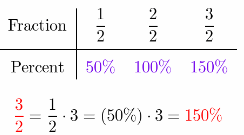# Texas Go Math Grade 6 Lesson 9.1 Answer Key Understanding Percent

Refer to our Texas Go Math Grade 6 Answer Key Pdf to score good marks in the exams. Test yourself by practicing the problems from Texas Go Math Grade 6 Lesson 9.1 Answer Key Understanding Percent.

## Texas Go Math Grade 6 Lesson 9.1 Answer Key Understanding Percent

Using a Grid to Model Percents

A percent is a ratio that compares a number to 100. The symbol % is used to show a percent.
17% is equivalent to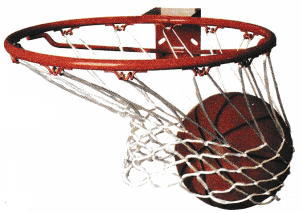• $$\frac{17}{100}$$ • 17 to 100 • 17:100

The free-throw ratios for three basketball players are shown.

Player 1: $$\frac{17}{25}$$
Player 2: $$\frac{33}{50}$$
Player 3: $$\frac{15}{20}$$

(A) Rewrite each ratio as a number compared to 100. Then shade the grid to represent the free-throw ratio.(B) Which player has the greatest free-throw ratio? ____________________
How is this shown on the grids? ____________________

(C) Use a percent to describe each player’s free-throw ratio. Write the percents in order from least to greatest.

(D) How did you determine how many squares t0 shade on each grid?

Connecting Fractions and Percents

You can use a percent bar model to model a ratio expressed as a fraction and to find an equivalent percent.

(A) Use a percent bar model to find an equivalent percent for $$\frac{1}{4}$$.
Draw a model to represent 100 and divide it into fourths. Shade $$\frac{1}{4}$$.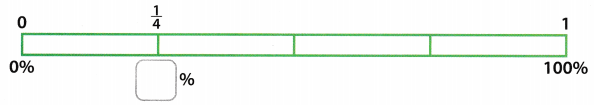$$\frac{1}{4}$$ of 100 = 25, so $$\frac{1}{4}$$ of 100% = _________________
Tell which operation you can use to find $$\frac{1}{4}$$ of 100.
Then find $$\frac{1}{4}$$ of 100%. _________________

(B) Use a percent bar model to find an equivalent percent for $$\frac{1}{3}$$.
Draw a model and divide it into thirds. Shade $$\frac{1}{3}$$.$$\frac{1}{3}$$ of 100 = 33$$\frac{1}{3}$$, so $$\frac{1}{3}$$ of 100% = _________________
Tell which operation you can use to find $$\frac{1}{3}$$ of 100.
Then find $$\frac{1}{3}$$ of 100%. _________________

Reflect

Question 1.
Critique Reasoning Jo says she can find the percent equivalent of $$\frac{3}{4}$$ by multiplying the percent equivalent of $$\frac{1}{4}$$ by 3. How can you use a percent bar model to support this claim?
The fraction $$\frac{3}{4}$$ can be written as 3 × $$\frac{1}{4}$$. This implies that the fraction is product of a unit fraction and 3. In percentage expression; $$\frac{3}{4}$$ = 25% and $$\frac{3}{4}$$ = 3(25%) = 75%.

Use a benchmark to find an equivalent percent for each fraction.

Question 2.
$$\frac{9}{10}$$ _______________
Write $$\frac{9}{10}$$ as a multiple of a benchmark fraction.
$$\frac{9}{10}$$ = 9 × $$\frac{1}{10}$$
Think: $$\frac{9}{10}$$ = $$\frac{1}{10}+\frac{1}{10}+\frac{1}{10}+\frac{1}{10}+\frac{1}{10}+\frac{1}{10}+\frac{1}{10}+\frac{1}{10}+\frac{1}{10}$$
Find an equivalent percent for $$\frac{1}{10}$$
$$\frac{1}{10}$$ = 10%
Multiply
$$\frac{9}{10}$$ = 9 × $$\frac{1}{10}$$ = 9 × 10% = 90%

Question 3.
$$\frac{2}{5}$$ _______________
Solution to this example is given below
$$\frac{2}{5}$$ = 2 ÷ 5 = 0.4 (Divide the numerator by the denominator)
0.4 = 40% (Move the decimal point 2 places to the right)
Final solution = 40%

Question 4.
64% of the animals at an animal shelter are dogs. About what fraction of the animals at the shelter are dogs?
Find a fraction equivalent for 64%
64% = $$\frac{64}{100}$$
64 % = $$\frac{64 \div 4}{100 \div 4}$$ (Divide numerator and denominator by 4)
64% = $$\frac{16}{25}$$
Final solution = $$\frac{16}{25}$$
$$\frac{16}{25}$$ of the animals in the shelter are dogs.

Question 1.
Shade the grid to represent the ratio $$\frac{9}{25}$$. Then find a percent equivalent to the given ratio.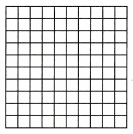Given fraction:
$$\frac{9}{25}$$

Multiply the given fraction with 100% to convert it to an equivalent percentage, therefore:
= $$\frac{9}{25}$$ × 100%
Evaluate:
= 36%

Here this implies that 36 of the 100 boxes must be shaded, therefore:Shade 36 boxes in the 100 boxes diagram shown.

Question 2.
Use the percent bar model to find the missing percent.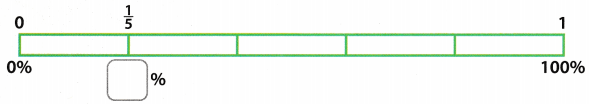Given fraction: $$\frac{1}{5}$$

Multiply the given fraction with 100% to convert it to an equivalent percentage, therefore:
= $$\frac{1}{5}$$ × 100%
Evaluate: = 20%

$$\frac{1}{5}$$ = 20%

Identify a benchmark you can use to find an equivalent percent for each ratio. Then find the equivalent percent.

Question 3.Write $$\frac{6}{10}$$ as a multiple of a benchmark fraction.
$$\frac{6}{10}$$ = 6 × $$\frac{1}{10}$$
Think: $$\frac{6}{10}$$ = $$\frac{1}{10}+\frac{1}{10}+\frac{1}{10}+\frac{1}{10}+\frac{1}{10}+\frac{1}{10}$$
Find an equivalent percent for $$\frac{1}{10}$$
$$\frac{1}{10}$$ = 10%
Multiply
$$\frac{6}{10}$$ = 6 × $$\frac{1}{10}$$ = 6 × 10% = 60%

Question 4.Solution to this example is given below
$$\frac{2}{4}$$ = 2 ÷ 4 = 0.50 (Divide the numerator by the denominator.)
0.50 = 50% (Move the decimal point 2 places to the right)
Final solution = 50%

Question 5.Solution to this example is given below
$$\frac{4}{5}$$ = 4 ÷ 5 = 0.80 (Divide the numerator by the denominator.)
0.80 = 80% (Move the decimal point 2 places to the right)
Final solution = 80%

Question 6.
41% of the students at an art college want to be graphic designers. About what fraction of the students want to be graphic designers?
Note that 41% is close to the benchmark 40% .
Find a fraction equivalent for40%
40% = $$\frac{2}{5}$$
Final solution = $$\frac{2}{5}$$
Approximately $$\frac{2}{5}$$ of the students want to be graphic designers.

Essential Question Check-In

Question 7.
How do you write a ratio as a percent?
First we can write the given ratio as a fraction, and then expand or reduce that fraction so that it has the denominator of 100. Then the number in the numerator is the percent equivalent to the starting ratio.

For example, let our ratio be 3 : 10
3 : 10 = $$\frac{3}{10}=\frac{3 \cdot 10}{10 \cdot 10}=\frac{30}{100}$$
so 30% is equivalent to 3 : 10

Generally, if the given ratio is a : b, we do the same thing:
a : b = $$\frac{a}{b}$$ = $$\frac{a \cdot \frac{100}{b}}{b \cdot \frac{100}{b}}$$ = $$\frac{\frac{100 \cdot a}{b}}{100}$$
So $$\left(\frac{100 \cdot a}{b}\right)$$ % is equivalent to a : b

We divide the first term of the ratio with the second, and multiply that number by 100(%)

Shade the grid to represent the ratio. Then find the missing number.

Question 8.Given fraction:
$$\frac{23}{50}$$

Multiply the given fraction with 100% to convert ¡t to an equivalent percentage, therefore:
= $$\frac{23}{50}$$ × 100%
Evaluate:
= 46%

Here this implies that 46 of the 100 boxes must be shaded, therefore: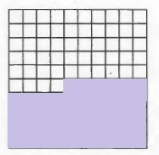Shade 46 of the 100 boxes shown.

Question 9.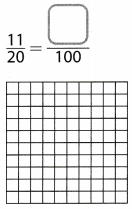Given fraction:
$$\frac{11}{20}$$

Multiply the given fraction with 100% to convert ¡t to an equivalent percentage, therefore:
= $$\frac{11}{20}$$ × 100%
Evaluate:
= 55%

Here this implies that 55 of the 100 boxes must be shaded, therefore:Shade 55 of the 100 boxes shown.

Question 10.
Mark wants to use a grid like the ones in Exercises 8 and 9 to model the percent equivalent of the fraction $$\frac{2}{3}$$. How many grid squares should he shade? What percent would his model show?
There are 100 squares on the grid.
To find how many squares should he shade, multiply 100 by $$\frac{2}{3}$$:
$$\frac{2}{3}$$ · 100 = 66.67 ≈ 67 = 67%

Question 11.
The ratios of saves to the number of save opportunities are given for three relief pitchers: $$\frac{9}{10}, \frac{4}{5}, \frac{17}{20}$$. Write each ratio as a percent. Order the percents from least to greatest.
$$\frac{9}{10} \cdot \frac{10}{10}$$ = $$\frac{90}{100}$$ = 90%
$$\frac{4}{5} \cdot \frac{20}{20}$$ = $$\frac{80}{100}$$ = 80%
$$\frac{17}{20} \cdot \frac{5}{5}$$ = $$\frac{85}{100}$$ = 85%
80% < 85% < 90%

Circle the greater quantity.

Question 12.
$$\frac{1}{3}$$ of a box of Corn Krinkles
50% of a box of Corn Krinkles
50% of a box is the same as $$\frac{1}{2}$$ box, and we know that
$$\frac{1}{2}=\frac{3}{6}$$ > $$\frac{2}{6}=\frac{1}{3}$$
So 50% of a box is the greater quantity.

Question 13.
30% of your minutes are used up
$$\frac{1}{4}$$ of your minutes are used up
Solution to this example is given below
$$\frac{1}{4}$$ = 1 ÷ 4 = 0.25 (Divide the numerator by the denominator.)
0.25 = 25% (Move the decimal point 2 places to the right.)
Since 25% < 30% , therefore: $$\frac{1}{4}$$ < 30%
30% = Final solution
30% of minutes is a greater quantity.

Question 14.
Multiple Representations Explain how you could write 35% as the sum of two benchmark percents or as a multiple of a percent.
Given percentage: = 35%

Divide the given percentage with 100% to convert it to an equivalent fraction, therefore:
= $$\frac{35 \%}{100 \%}$$
Evaluate:
= $$\frac{7}{20}=\frac{3}{10}+\frac{1}{20}$$
35% is equal to $$\frac{7}{20}$$ which is a sum of $$\frac{3}{10}+\frac{1}{20}$$. The benchmarks here are $$\frac{1}{10}$$ and $$\frac{1}{20}$$.

35% as a multiple of a percent is 7(5%).

Question 15.
Use the percent bar model to find the missing percent.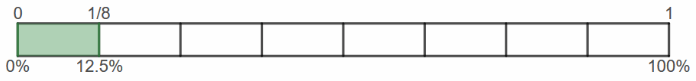The percent bar is divided into 8 blocks of the same size.
So, just as we calculate that one block is 1 ÷ 8 = $$\frac{1}{8}$$ of the whole bar, we can do the same thing with percentages.
One block of the percent bar equals 100% ÷ 8 = 12.5% of the whole bar.

Question 16.a. What is the total number of songs Carl downloaded last year?
Evaluate the total number of songs by taking the sum of the songs downloaded in each category. Therefore: 15 + 20 + 5 + 10 = 50.

b. What fraction of the songs were country? Find the fraction for each type of song. Write each fraction in simplest form and give its percent equivalent.
Find the fraction for each type of song downloaded by dividing its share in the collection by the total number of songs downloaded. Divide out the common factor to write as a fraction is simplest form? therefore:
Fraction of Country songs = $$\frac{15}{50}$$ = $$\frac{3}{10}$$
Fraction of Rock songs = $$\frac{20}{50}$$ = $$\frac{2}{5}$$
Fraction of Classical songs = $$\frac{5}{50}$$ = $$\frac{1}{10}$$
Fraction of World songs = $$\frac{10}{50}$$ = $$\frac{1}{5}$$

Multiply each of the given fraction with 100% to convert it to an equivalent percentage, therefore:
Fraction of Country songs = $$\frac{3}{10}$$ × 100% = 30%
Fraction of Rock songs = $$\frac{2}{5}$$ × 100% = 40%
Fraction of Classical songs = $$\frac{1}{10}$$ × 100% = 10%
Fraction of World songs = $$\frac{1}{5}$$ × 100% = 20%

H.O.T. Focus On Order Thinking

Question 17.
Critique Reasoning Marcus bought a booklet of tickets to use at the amusement park. He used 50% of the tickets on rides, $$\frac{1}{3}$$ of the tickets on video games, and the rest of the tickets in the batting cage. Marcus says he used 10% of the tickets in the batting cage. Do you agree? Explain.
The fraction $$\frac{1}{3}$$ is equal to 33.$$\overline{3}$$%. This means that the percentage of tickets used in batting cage are equal to 100% – 50% – 33.$$\overline{3}$$% 16.$$\overline{6}$$% ≠ 10%. Therefore, Marcus is incorrect in his reasoning.

Question 18.
Look for a pattern Complete the table.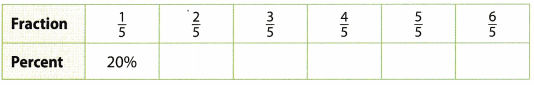a. Analyze Relationships What is true when the numerator and denominator of the fraction are equal? What is true when the numerator is greater than the denominator?
$$\frac{x}{x}$$ = 1, for any number x
In the context of this table, i.e. it we assume that we are dealing with positive percentages, then
a > b => $$\frac{a}{b}$$ > 1 = 100%
However, if we are dealing with negative numbers as well, this is not true.
If the numerator is greater than the denominator, then we can write the numerator as “denominator + some positive number”
Then we havewhich is less/greater than 1 = 100% if the denominator is negative/positive number.
See strict proof at the bottom.

(a) let our fraction be $$\frac{a}{b}$$, where a > b
That is equivalent to a – b > 0
Now, let’s write c = a – b > 0
Now we haveFinally, since c > 0, the answer to the question depends on a
1) It a is positive, then $$\frac{c}{a}$$ > 0 ⇒ 1 + $$\frac{c}{a}$$ > 1 = 100%
2) If a is negative, then $$\frac{c}{a}$$ < 0 ⇒ 1 + $$\frac{c}{a}$$ < 1 = 100%
For example, 1 > – 4 and
$$\frac{1}{-4}$$ = – 0.25 = – 25% < 100% = 1
also, – 3 > – 8, but
$$\frac{-3}{-8}=\frac{3}{8}$$ = 0.375 = 37.5% < 100% = 1

b. Justify Reasoning What is the percent equivalent of $$\frac{3}{2}$$? Use a pattern like the one in the table to support your answer.